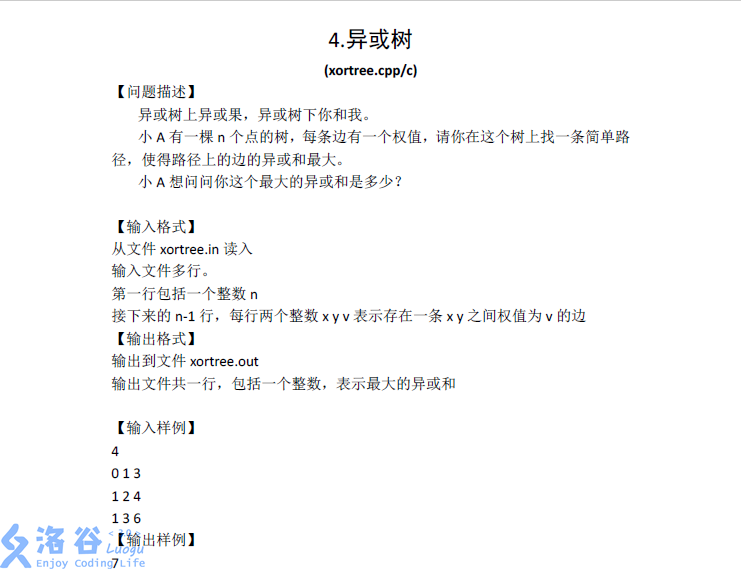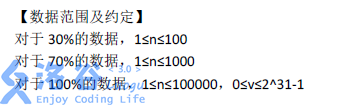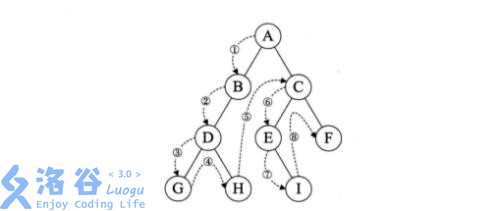# 题面：# 解析：

## 例图e.g.1:G——E:

A——E的路径异或和：AC^CE;

e.g.2:G——H(换两个有重复的试试？

A——H的路径异或和：AB^BD^DH;

？？？

1^1=0;

1^0=1;

0^1=1;

0^0=0;

### 字典树？

PS:左下角被挡住的两个单词是inn和int

# 代码

#include <stdio.h>
#include <cstdlib>
#include <cstring>
#include <cmath>
#include <iostream>
#include <algorithm>
#define maxn 100010

using namespace std;

struct edge{
int next,to,val;
}e[maxn*2];

int point[maxn],cnt,n,xorval[maxn];
int t[maxn*32],root,size,ans;
bool vis[maxn];

{
e[++cnt].next=point[x];
e[cnt].to=y;
e[cnt].val=val;
point[x]=cnt;
}

void dfs(int x)
{
vis[x]=1;
for(int i=point[x];i;i=e[i].next)
{
int y=e[i].to;
if(!vis[y])
{
xorval[y]=xorval[x]^e[i].val;
dfs(y);
}
}
}

void insert(int val)
{
int x=root;
for(int i=30;i>=0;i--)
{
int tmp=(val>>i)&1;
if(t[x][tmp]==-1) t[x][tmp]=++size;
x=t[x][tmp];
}
}

void init()
{
memset(t,-1,sizeof(t));
memset(e,0,sizeof(e));
memset(point,0,sizeof(point));
memset(vis,0,sizeof(vis));
memset(xorval,0,sizeof(xorval));
ans=cnt=size=root=0;
}

void query(int val)
{
int x=root;
int sum=0;
for(int i=30;i>=0;i--)
{
int tmp=(val>>i)&1;
if(t[x][tmp^1]==-1)
x=t[x][tmp];
else
{
x=t[x][tmp^1];
sum+=(1<<i);
}
}
ans=max(ans,sum);
}

int main()
{
//freopen("xortree.in","r",stdin);
//freopen("xortree.out","w",stdout);
while(scanf("%d",&n)!=EOF)
{
init();
for(int i=1;i<n;i++)
{
int x,y,z;
scanf("%d%d%d",&x,&y,&z);
x++;
y++;
}